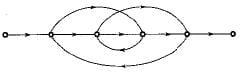The signal flow graph shown below has M number of forward path and N number of individual loops.Q. ​What are the values of M and N ?
• a)
M= 3 and N= 4
• b)
M = 4 and N = 6
• c)
M = 4 and N = 4
• d)
M = 2 and N = 6

### Related Test## Can you answer this doubt?

People are searching for an answer to this question.